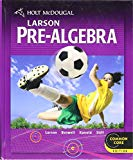# Suppose 36 participants complete an experiment where ads are presented subliminally during a task (e.g., Coke ads are flashed at very fast rates during movie ads). Participants are then given a recognition test for images of the ads, where two images are presented and participants must choose which of the two was presented earlier. If participants are able to process the subliminal ads when they are first presented, then their performance should be above chance (50%). This is what the researcher predicts. However, if the ads were not processed and the participants are only guessing, then their performance should be similar to chance.  Answer the following questions.   a) State the alternative and null hypotheses for this study. b) The sample mean for this study was 67% with a standard deviation of 12%. Calculate the sample tscore for this study. c) What decision should the researcher make about the null hypothesis in this study? (You can use Table 9.1 to find the tcrit.) What can the researcher conclude about their prediction from this decision?

Question

Suppose 36 participants complete an experiment where ads are presented subliminally during a task (e.g., Coke ads are flashed at very fast rates during movie ads). Participants are then given a recognition test for images of the ads, where two images are presented and participants must choose which of the two was presented earlier. If participants are able to process the subliminal ads when they are first presented, then their performance should be above chance (50%). This is what the researcher predicts. However, if the ads were not processed and the participants are only guessing, then their performance should be similar to chance.  Answer the following questions.

a) State the alternative and null hypotheses for this study.

b) The sample mean for this study was 67% with a standard deviation of 12%. Calculate the sample tscore for this study.

c) What decision should the researcher make about the null hypothesis in this study? (You can use Table 9.1 to find the tcrit.) What can the researcher conclude about their prediction from this decision?

Expert Solution

### Want to see the full answer?

Check out a sample Q&A hereStudents who’ve seen this question also like:Holt Mcdougal Larson Pre-algebra: Student Edition 2012
1st Edition
ISBN: 9780547587776
Author: HOLT MCDOUGAL
Publisher: HOLT MCDOUGAL